Function Repository Resource:

# ThickLensManipulate

Generate a graphic in which the thick-lens parameters can be manipulated dynamically

Contributed by: Julián Laverde
Jason Martinez
 ResourceFunction["ThickLensManipulate"][] returns a manipulable graphic of the ray diagram of a thick lens. ResourceFunction["ThickLensManipulate"][assoc] returns a graphic with the corresponding parameters in the Association assoc.

## Details and Options

The available type thick lenses are biconvex, plano-convex, meniscus, plano-concave, and biconcave.
ResourceFunction["ThickLensManipulate"] follows the sign convention for thick lenses from Hecht, Optics, Pearson, 2015:
 Object distance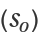(+) real object (-) virtual object Image distance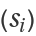(+) real image (-) virtual image Object size (+) erect object (-) inverted object Image size (+) erect image (-) inverted image Magnification (M) (+) erect image (-) inverted image
The linear magnification factor of the system is given by the equation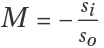defined as the ratio of the size of the image compared to the size of the object.
ResourceFunction["ThickLensManipulate"] uses the lensmaker’s equation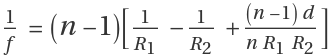to calculate the focal length f of a thick lens in air, where n is the lens refractive index, and d, R1 and R2 are the lens thickness, and radii of curvature of the lens surfaces respectively, as shown in the following figure: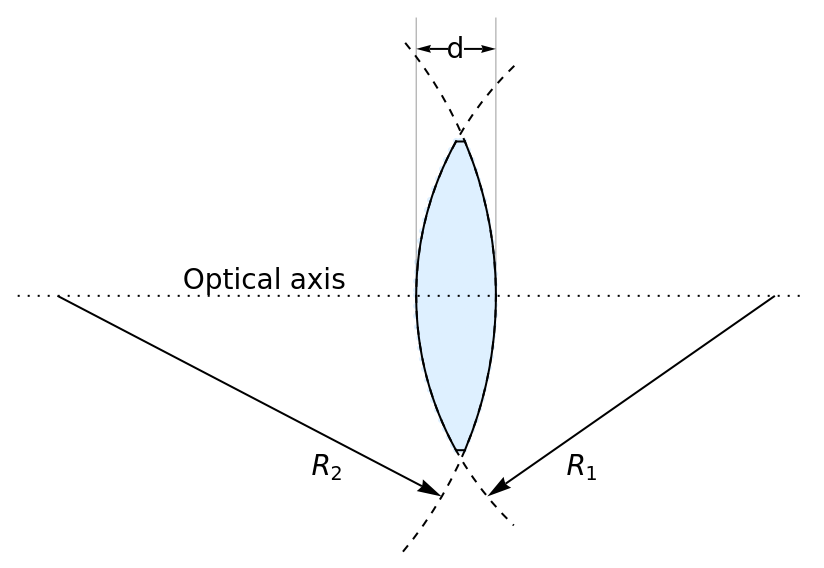The arrow in the middle of the rays represents the direction of light and dotted lines the projection of the rays.
Real images and virtual images are represented by a thick-line arrow and a dotted-line arrow, respectively.
In ResourceFunction["ThickLensManipulate"][assoc], the Association assoc can contain the following elements:
 "LensThickness" 5 initial lens thickness "Radius1" 3 initial radius of the first surface encountered by light, as a multiple of lens thickness "Radius2" -3 initial radius of the second surface encountered by light, as a multiple of lens thickness "RefractiveIndex" 1.5 initial refractive index of the lens "ObjectSize" 0.5 initial object size "ObjectDistance" FocalLength initial object position
"LensThickness" and "ObjectDistance" can be a positive numbers or quantities of length.
"RefractiveIndex" can be a number in the range[1,3].
"Radius2" can be Infinity for plano-concave and plano-convex lenses.
"ObjectSize" can be a number in the range [0,1].
Quantity inputs should have units of length. Results and values in sliders will be shown in the most common unit among the inputs.
ResourceFunction["ThickLensManipulate"][] takes the options of Graphics and the following:
 "RayColor" Automatic specify the colors of the rays "LensColor" Automatic specify the color of the lens "ArrowColor" Automatic specify the colors of the object and image arrows "ShowEquations" True set the equations in the graphics object "ObjectDistanceRange" Automatic specify the range of "ObjectDistance" "ObjectSizeRange" Automatic specify the range of "ObjectSize" "Radius1Range" Automatic specify the range of "Radius1" "Radius2Range" Automatic specify the range of "Radius2" "RefractiveIndexRange" Automatic specify the range of "RefractiveIndex" "LensThicknessRange" Automatic specify the range of "LensThickness"
The copy values button copies the current values of "ObjectDistance", "FocalLength", "ImageDistance", and "Magnification" to the clipboard as an Association.

## Examples

### Basic Examples (1)

Get a manipulable thick lens ray diagram:

 In:=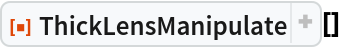Out=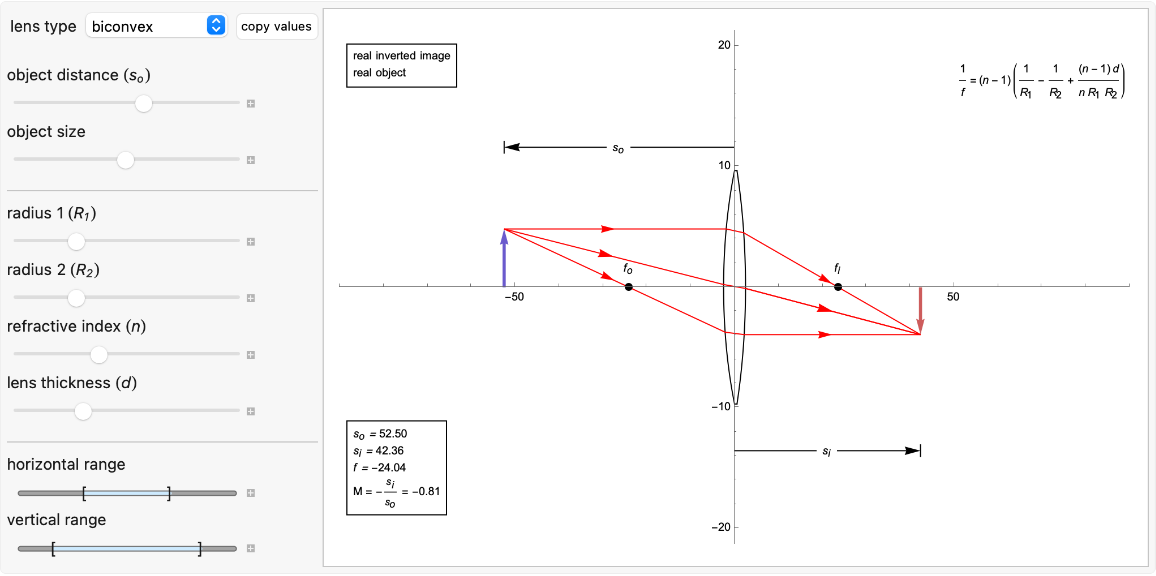### Scope (6)

Get the ray diagram for a biconcave lens:

 In:=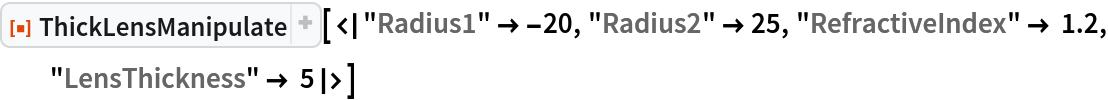Out=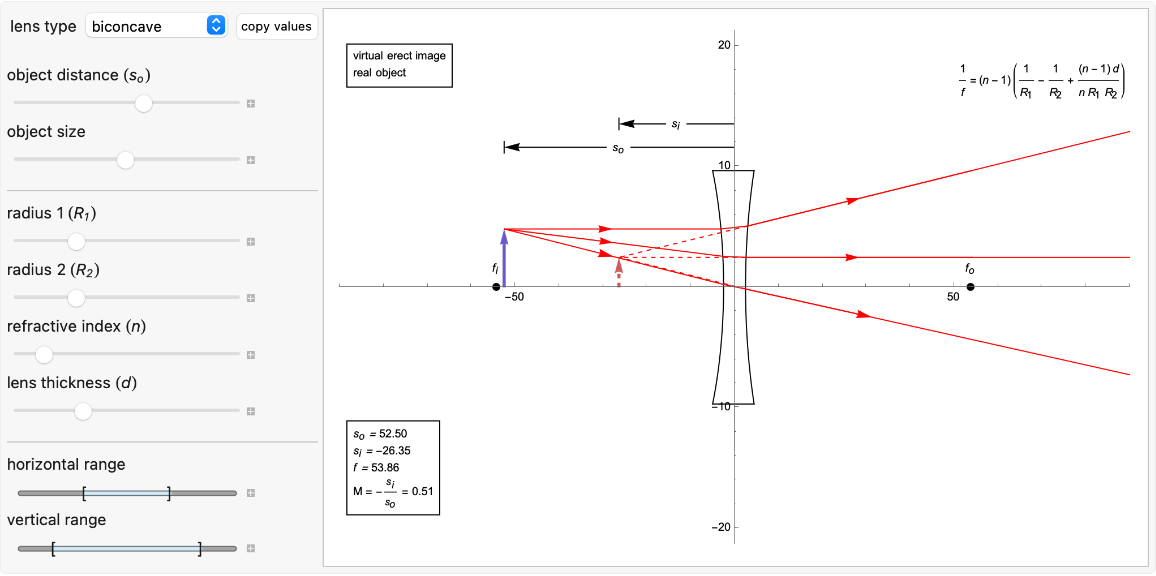Get the ray diagram for a plano-convex lens:

 In:=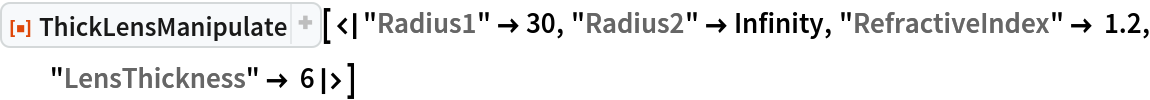Out=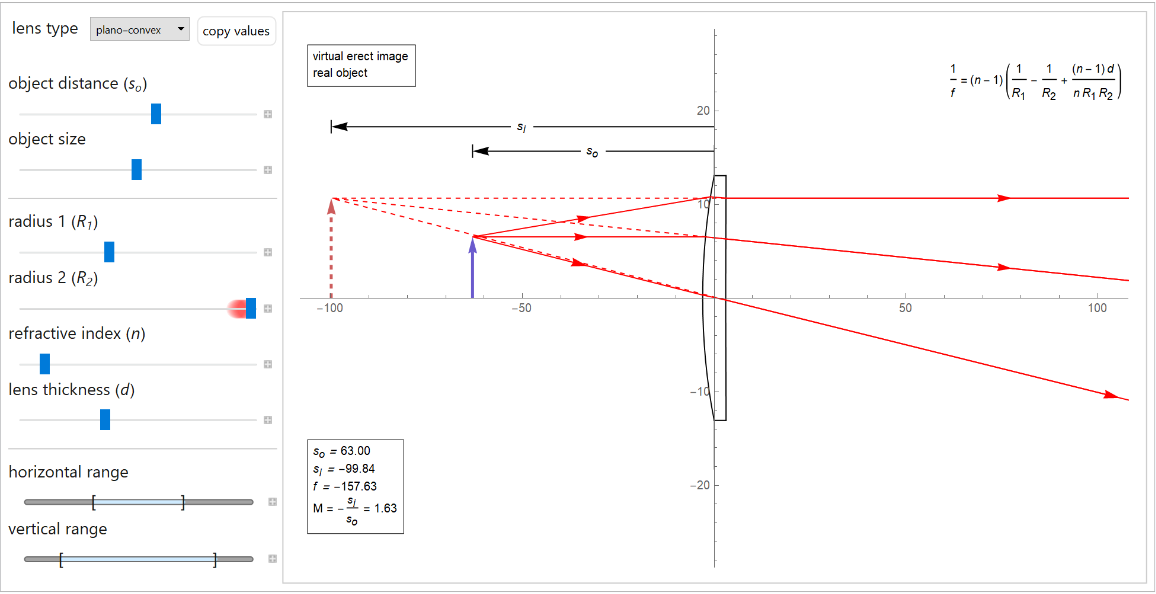Include inputs with quantities:

 In:=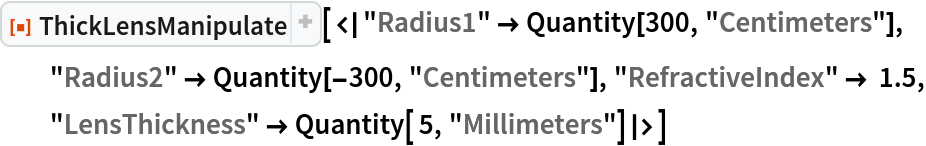Out=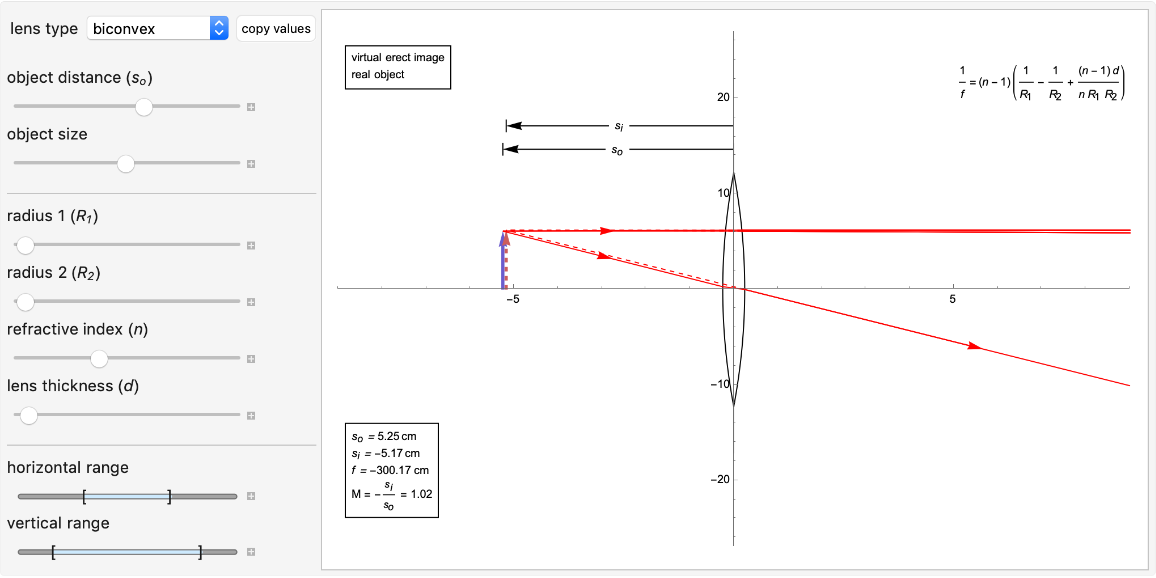Get the ray diagram for a plano-concave lens:

 In:=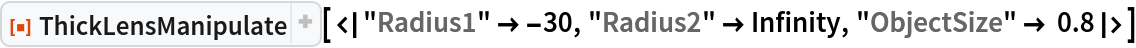Out=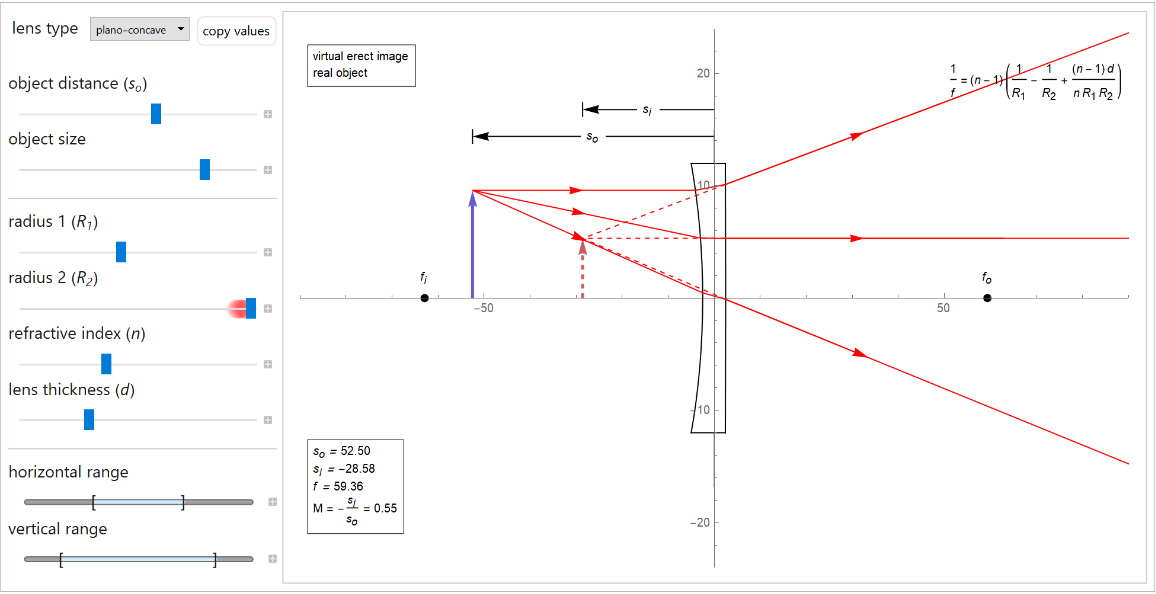Get the ray diagram for a negative meniscus lens:

 In:=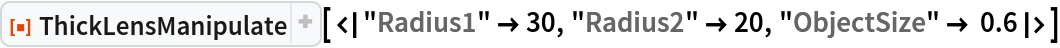Out=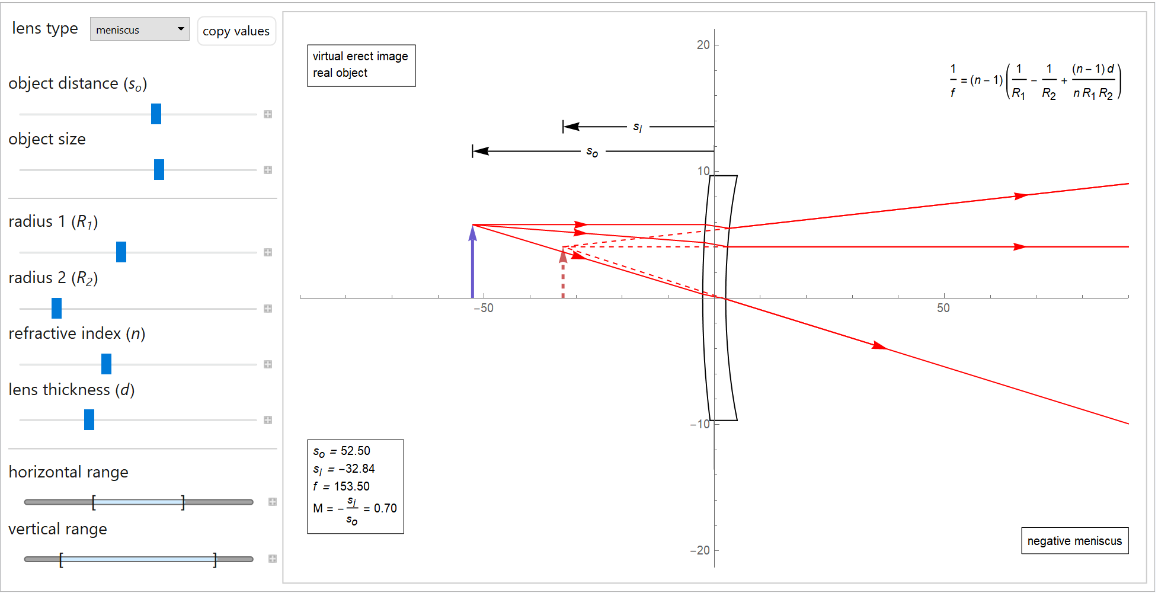Get the ray diagram for a positive meniscus lens:

 In:=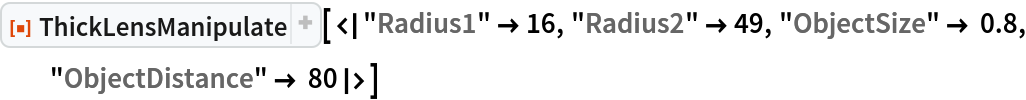Out=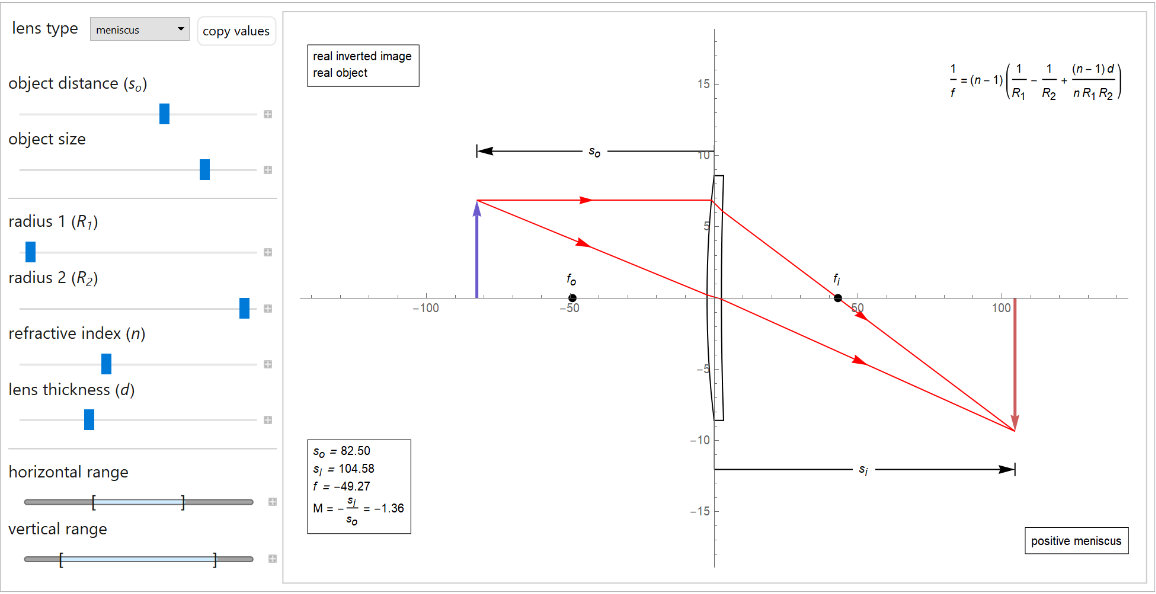### Options (10)

#### ShowEquations (1)

Set "ShowEquations" to False:

 In:=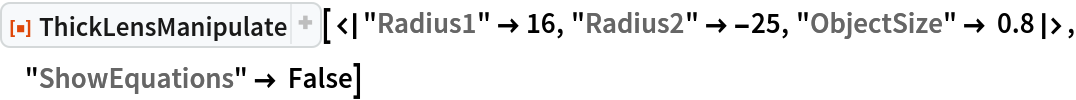Out=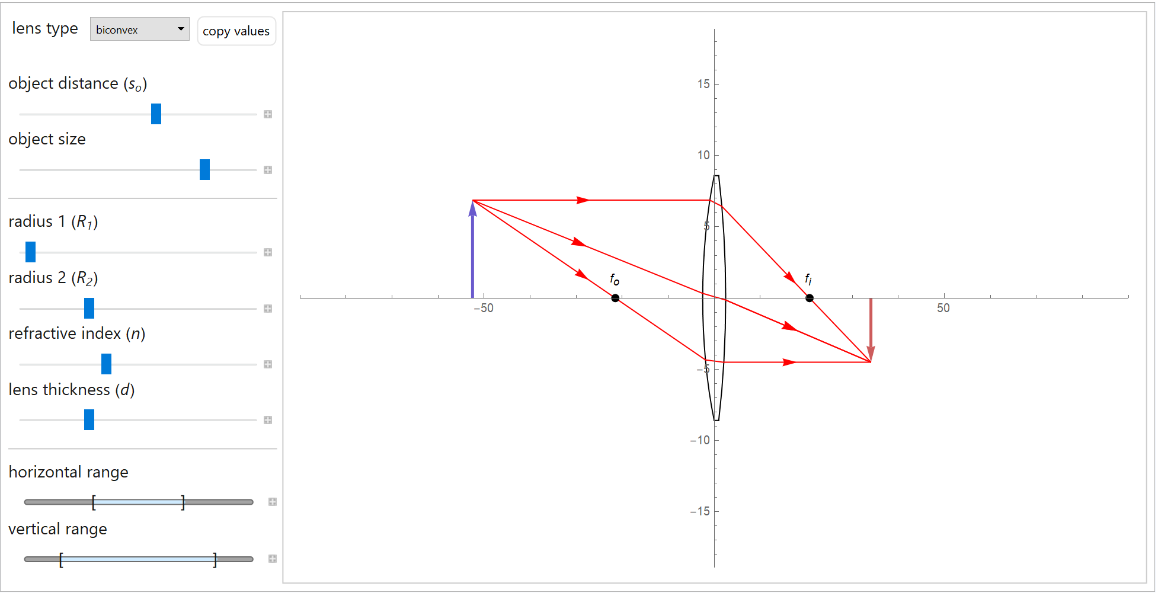#### RayColor (1)

Change the color of the rays:

 In:=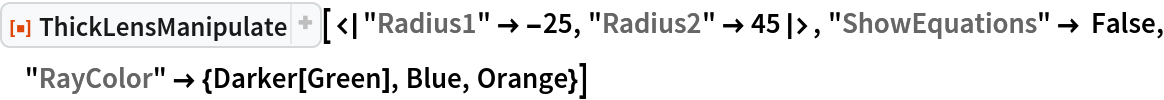Out=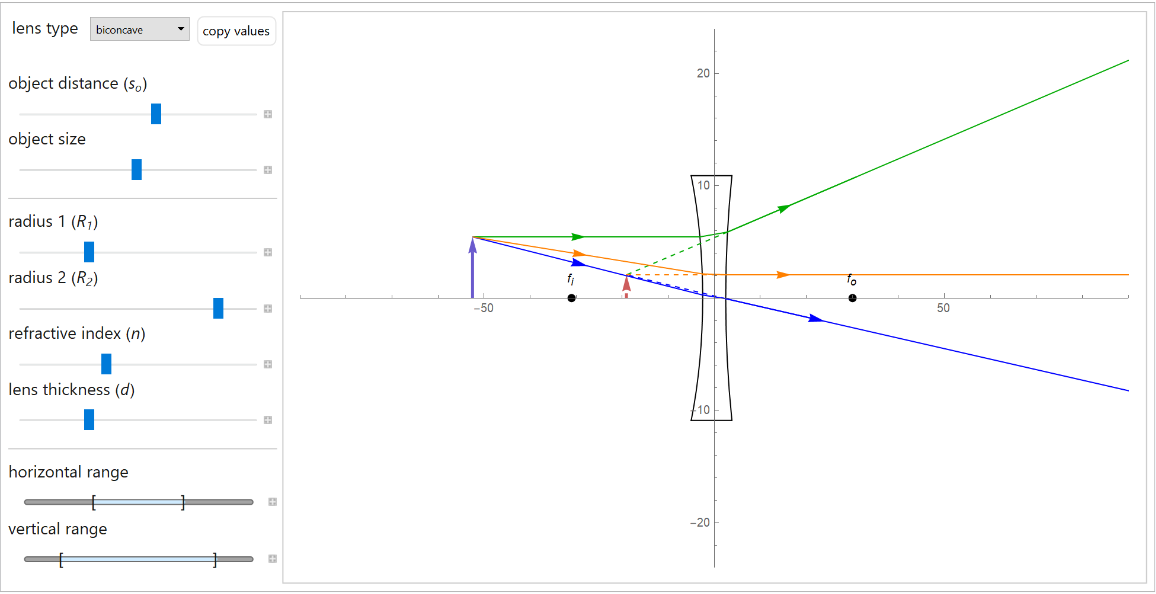#### ArrowColor (1)

Change the color of the object and virtual arrows:

 In:=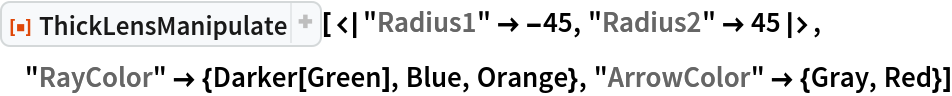Out=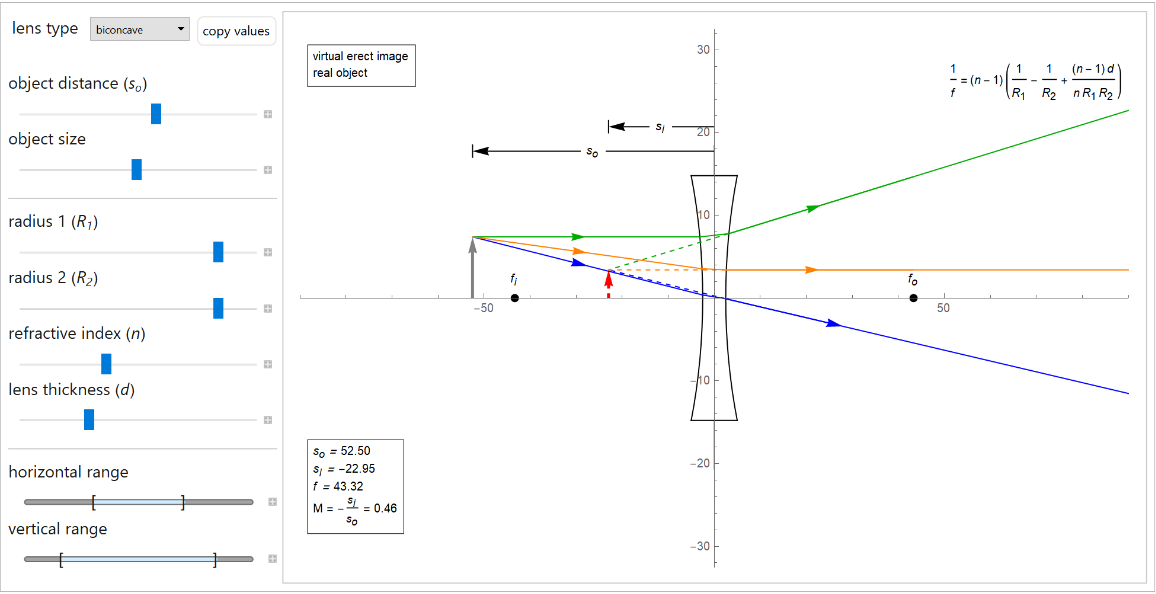#### LensColor (1)

Specify the color of the lens:

 In:=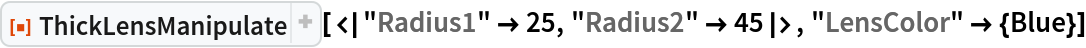Out=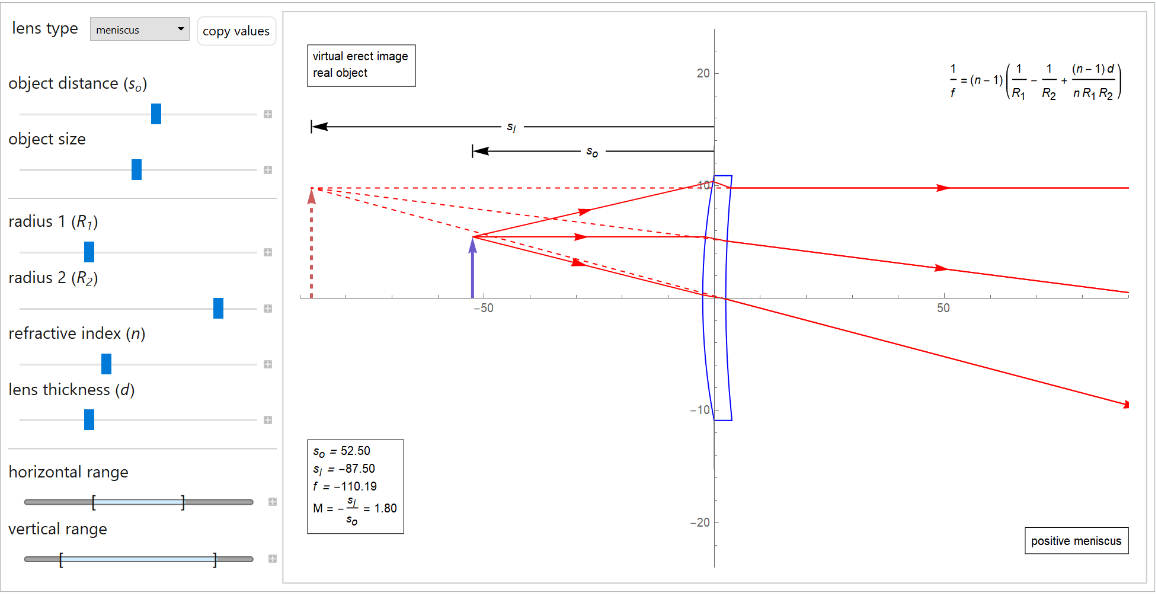#### ObjectDistanceRange (1)

Specify the range of "ObjectDistance":

 In:=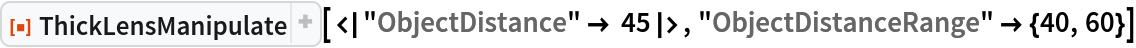Out=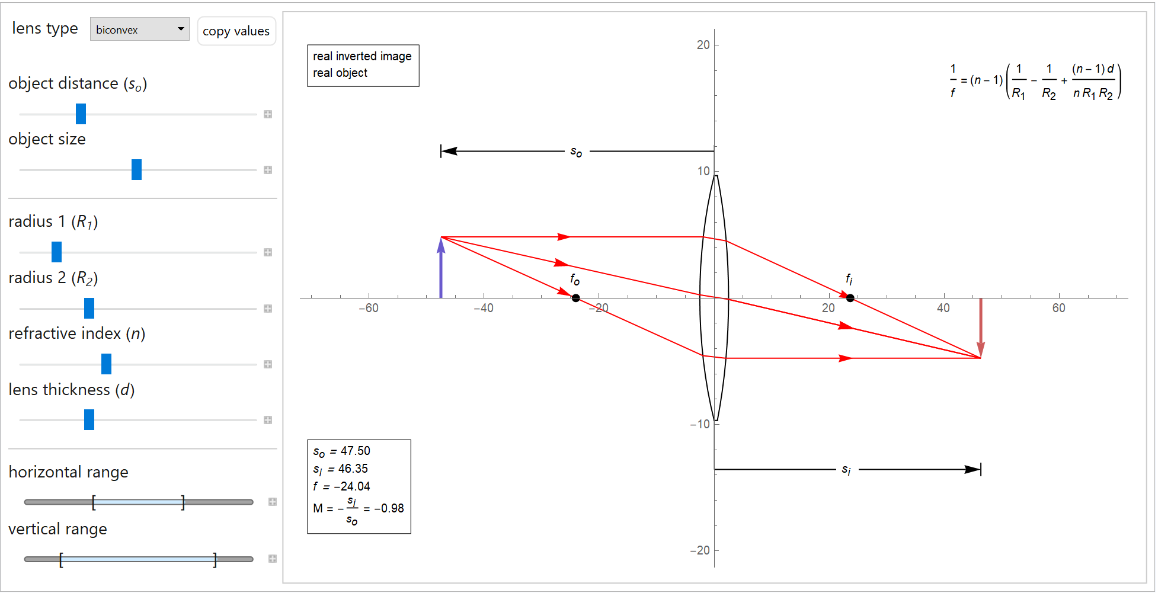#### ObjectSizeRange (1)

Specify the range of "ObjectSize":

 In:=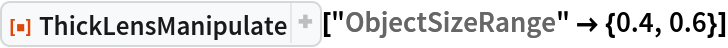Out=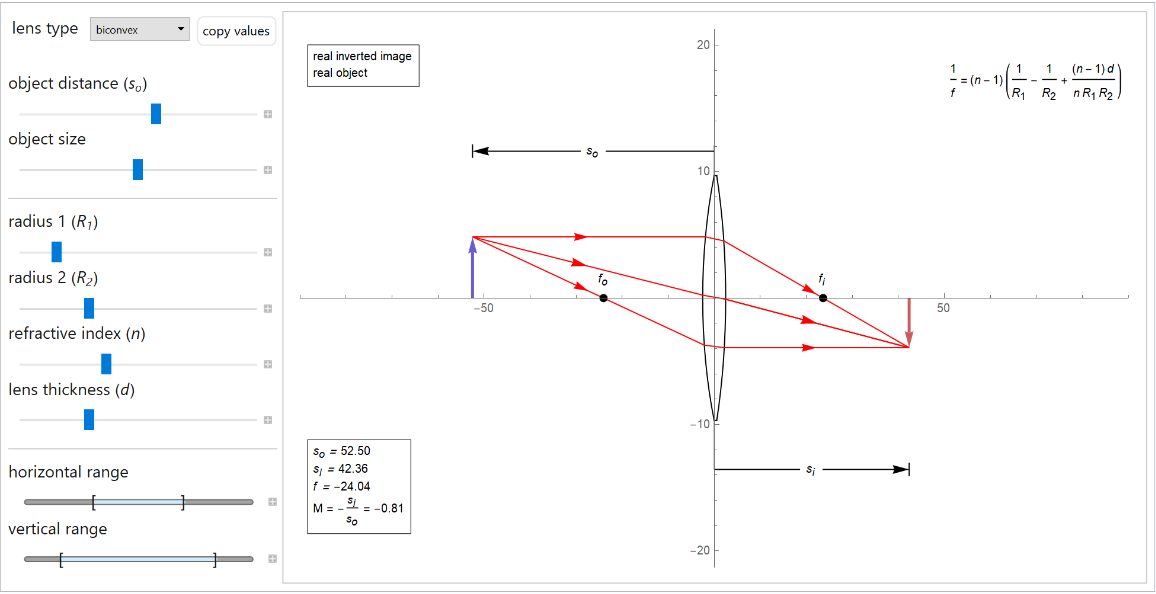In:=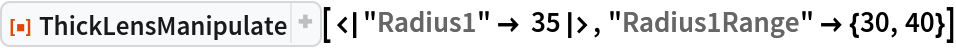Out=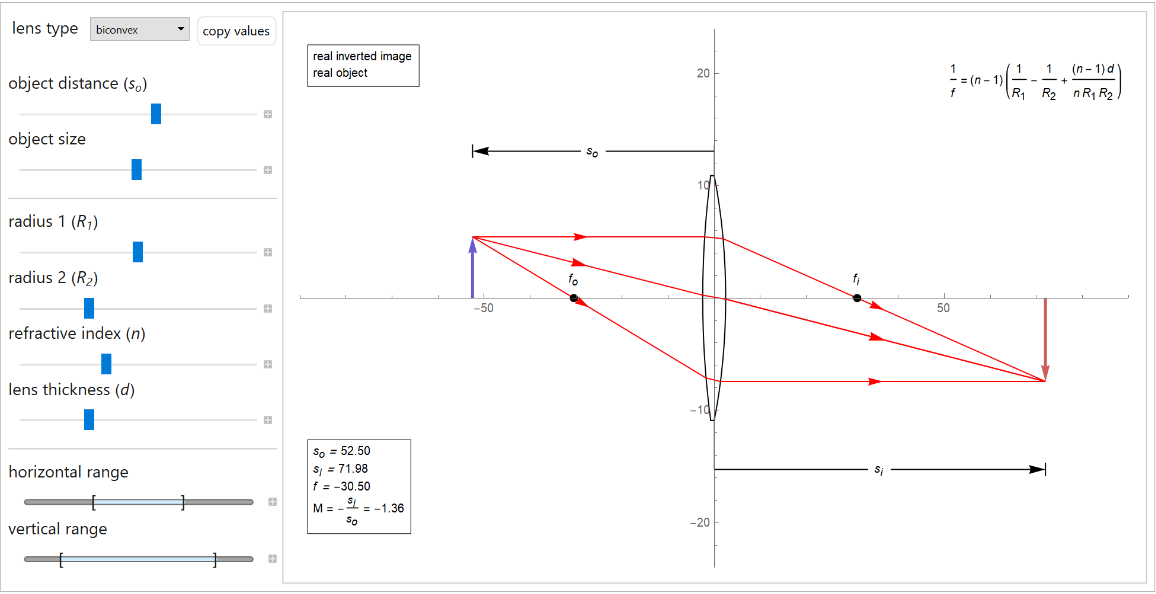In:=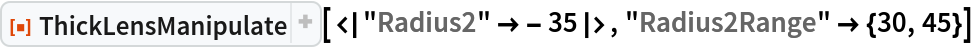Out=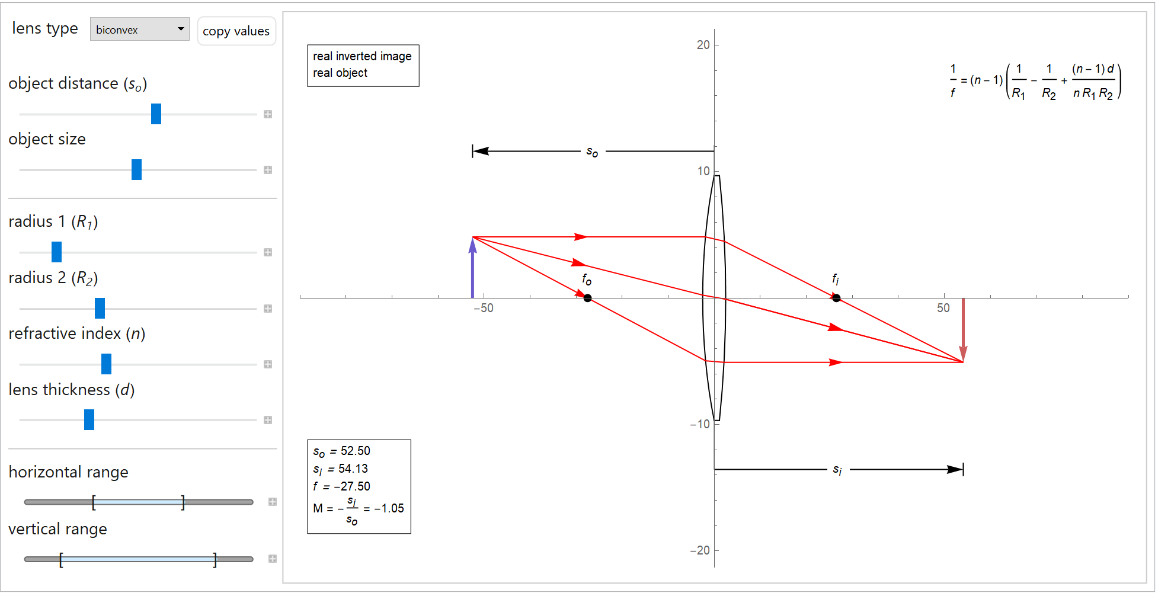#### RefractiveIndexRange (1)

Specify the range of "RefractiveIndex":

 In:=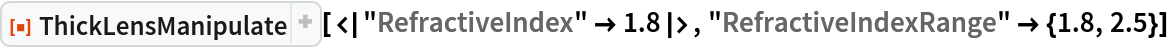Out=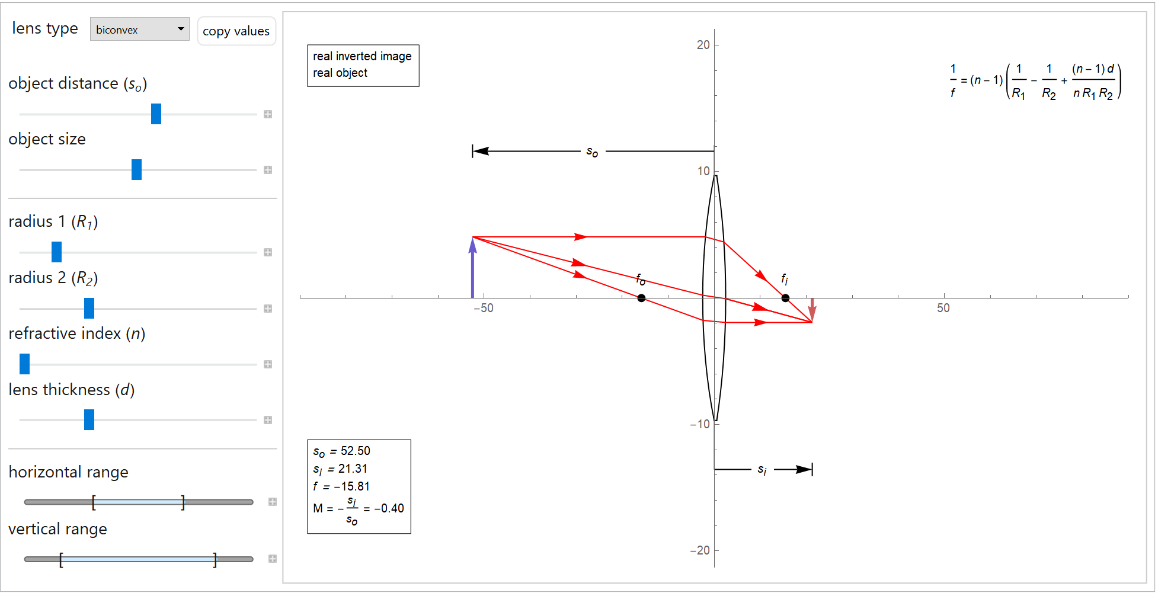#### LensThicknessRange (1)

Specify the range of "LensThickness":

 In:=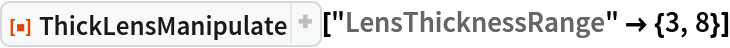Out=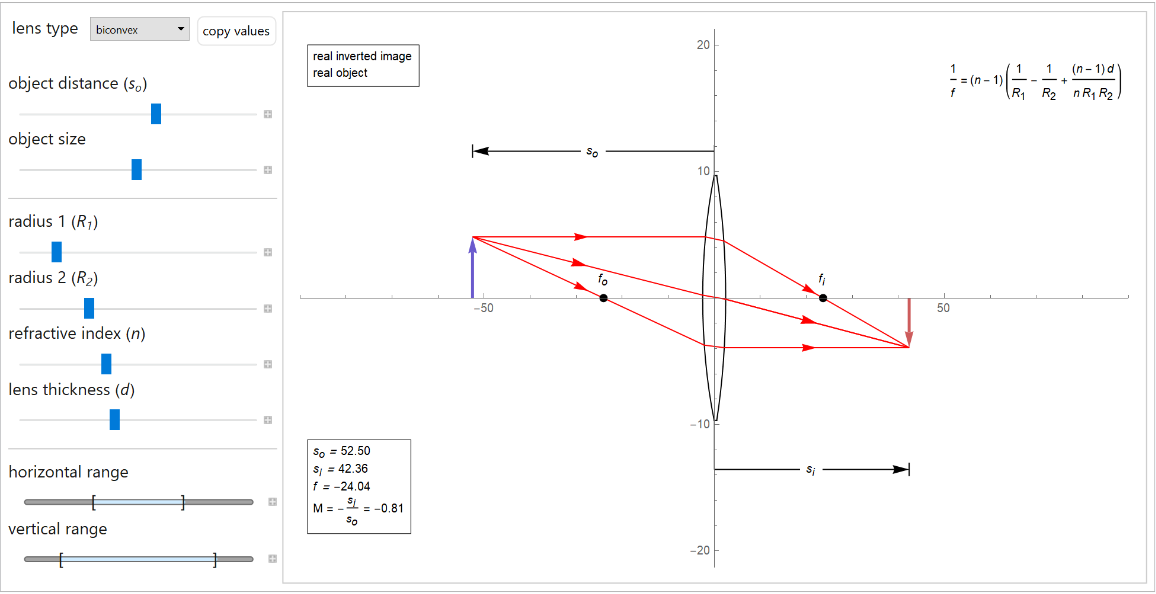## Publisher

WolframSpecialProjects

## Version History

• 1.1.0 – 12 September 2022
• 1.0.0 – 14 June 2021